Why WeightWatcher Works #AI - The Entrepreneurial Way with A.I.

## Tuesday, September 15, 2020

#A.I.

I am frequently asked, why does weightwatcher work ?

The weightwatcher tool uses power law fits to model the eigenvalue density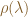$\rho(\lambda)$ of weight matrices of any Deep Neural Network (DNN).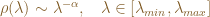$\rho(\lambda)\sim \lambda^{-\alpha},\;\;\;\lambda\in[\lambda_{min},\lambda_{max}]$

The average power-law exponent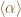$\langle\alpha\rangle$ is remarkably well correlated with the test accuracy when changing the number of layers and/or fine-tuning the hyperparameters. In our latest paper, we demonstrate this using a metaanalysis on hundreds of pre-trained models. This begs the question:

Why can we model the weight matrices of DNNs using power law fits ?

In theoretical chemistry and physics, we know that strongly correlated, complex systems frequently display power laws.

For example, in Tsallis’ theory of non-Extensive Statistical Mechanics, the sum of N correlated random variables is, under certain conditions, q-Gaussian distributed (i.e Heavy-Tailed); this is a generalization of the Central Limit Theorem (CLT) to correlated data. These kinds of complex systems display long-range space (and time) correlations. They are usually non-Markovian with long memories. They are non-ergodic and may even display multi-fractal characteristics. This is just one example; there are many.

In many machine learning and deep learning models, the correlations also display heavy / power law tails. After all, the whole point of learning is to learn the correlations in the data. Be it a simple clustering algorithm, or a very fancy Deep Neural Network, we want to find the most strongly correlated parts to describe our data and make predictions.

In my earlier days as a scientist, I worked on strongly correlated multi-reference ab initio methods for quantum chemistry. Here, the trick is to find the right correlated subspace to get a good low order description. I believe, in machine learning, the same issues arise.

##### Latent Semantic Analysis: a simple example of power law correlations

Take Latent Semantic Analysis (LSA). How do we select the Latent Space? We need to select the top-K components of the TF-IDF Term-Document matrix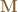$\mathbf{M}$. I believe this can be done by selecting those K eigenvalues that best fit a power law. Here is an example, using the scikit-learn 20newsgroups data:

We call ths plot the Empirical Spectral Density (ESD). This is just a histogram plot, on a log scale, of the eigenvalues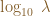$\log_{10}\; \lambda$ of the TF-IDF correlation matrix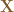$\mathbf{X}$. The correlation matrix is the square of the TF-IDF matrix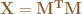$\mathbf{X}=\mathbf{M^{T}}\mathbf{M}$

and the eigenvalues of$\mathbf{X}$ are the singular values of$\mathbf{M}$, squared: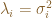$\lambda_{i}=\sigma_{i}^{2}$.

We fit the eigenvalues to a power law (PL) using the python powerlaw package, which implements a standard MLE estimator.

fit = powerlaw.fit(eigenvalues)


The fit selects the optimal xmin=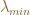$\lambda_{min}$ using a brute force search, and returns the best PL exponent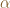$\alpha$, and the quality of the fit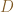$D$ (the KS-distance). The orange line displays the start of the power law tail, which contains the most strongly correlated eigenpairs.

We can evaluate the quality of the PL fit by comparing the ESD and the actual fit on log-log plot.

The blue solid line is the ESD on a log-log scale (or the PDF), and the blue dotted line is the PL fit. (The red solid line is the empirical CDF, and the red dotted line the fit). The PDF (blue) shows a very good linear fit up, except perhaps for largest eigenvalues,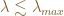$\lambda\lesssim\lambda_{max}$. Likewise, the CDF (red) shows a very good fit, up until the end of the tail. This is typical of power-law fits on real-world data and is usually best described as a Truncated Power Law (TPL), with some noise in the very far tail *(more on the noise in a future post). And the reported KS-distance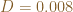$D=0.008$, which is exceptional.

We can get even more insight into the quality of the fit by examining how the PL method selected xmin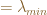$=\lambda_{min}$, the start of the PL. Below, we plot the KS-distance for each possible choice of xmin:

The optimization landscape is convex, with a clear global minimum at the orange line, which occurs at the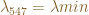$\lambda_{547}=\lambda{min}$. That is, there are 547 eigenpairs in the tail of the ESD displaying strong power-law behavior.

To form the Latent space, we select these largest 547 eigenpairs, to the right of the orange line, the start of the (truncated) power-law fit

##### Comparison with other K-selection methods

Typically, in unsupervised learning, one selects the top-K clusters, eigenpairs, etc. by looking at some so-called ‘elbow curve’, and identifying the K at the inflection point. We can make these plots too. A classic way is to plot the explained variance per eigenpair:

We see that the power-law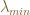$\lambda_{min}$, the orange line, occurs just to the right of the inflection point. So these two methods give similar results.

We can also plot localization ratios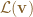$\mathcal{L}(\mathbf{v})$ in the same way, where the localization is defined as in our first paper: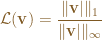$\mathcal{L}(\mathbf{v})=\dfrac{\Vert\mathbf{v}|\Vert_{1}}{\Vert\mathbf{v}|\Vert_{\infty}}$

def localization_ratio(v):
return np.linalg.norm(v, ord=1) / np.linalg.norm(v, ord=np.inf)


Again, we get an elbow curve, and the eigenvalue cutoff appears just to the right of the ‘elbow’:

##### Conclusion

I suspect that in these strongly correlated systems, the power law behavior really kicks it right at / before these inflection points. So we can find the optimal low-rank approximation to these strongly correlated weight matrices by finding that subspace where the correlations follow a power-law / truncated power law distribution. Moreover, we can detect, and characterize these correlations, by both the power-law exponent$\alpha$, and the quality of the fit D. And AFAIK, this has never been suggested before.

Ai

via https://www.AiUpNow.com

September 15, 2020 at 12:11AM by Charles H Martin, PhD, Khareem Sudlow